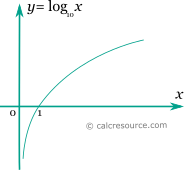## Decimal logarithm calculator

This tool evaluates the decimal logarithm of a number: . Enter the argument x below.

 x = Result: \log_{10}{x} =## Definitions

### General

The decimal (also called decadic) logarithm of a number x, is a power p so that 10p= x. In modern notation decimal logarithm is written as or lg(x). Any positive real number has a decimal logarithm. The decimal logarithm of 1 is 0, while the decimal logarithm of 0 approaches negative infinity.

The graph of the decimal logarithm function is shown in the figure below. It is a monotonic function.### Properties

The derivative of the decimal logarithm function is:

The integral of the decimal logarithm function is given by: